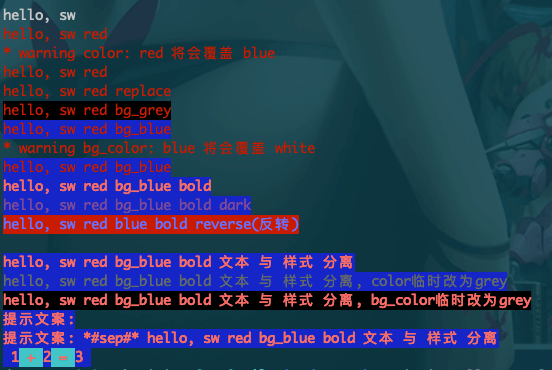## shede333 / SWTermColorPythonMIT

Explore and code with more than 5 million developers，Free private repositories ！：）
CLI终端输出彩色的文本, simple termcolor wrapper spread retract

• Python 100.0%
Notice: Creating folder will generate an empty file .keep, because not support in Git

# SWTermColor

## Install


pip install SWTermColor


## Example Modules:


from swtermcolor import SWTermColor
# 或者
from swtermcolor import ColorPrint
# 或者
from swtermcolor import PrintColor

# 注意：
# SWTermColor == ColorPrint == PrintColor, 三者都是等价的类名，根据喜好选用
SWTermColor().c_red().p("hello, SWTermColor")  # p 即 print的缩写
PrintColor().c_red().p("hello, PrintColor")
ColorPrint().c_red().p("hello, ColorPrint")

# 常用的几种打印方法：
SWTermColor("hello, sw red").c_red().p()  # 先设置文本，再设置效果
SWTermColor().c_red().p("hello, sw red")  # 先设置效果，再设置文本
print(SWTermColor("hello, sw red").c_red())  # 使用print方法打印
# 默认以", "为分隔符，打印所有文本
SWTermColor().c_red().p("hello", "sw", "red")
# 以" * "为分隔符，打印所有文本
SWTermColor().c_red().p("hello", "sw", "red", sep=" * ")

print(SWTermColor("hello, sw red").c_blue().c_red())
print(SWTermColor("hello, sw red bg_grey").c_red().b_grey())
SWTermColor("hello, sw red bg_blue").c_red().b_blue().p()
# 背景色：蓝色 覆盖了 白色，同时会打印一行覆盖警告
print(SWTermColor("hello, sw red bg_blue").c_red().b_white().b_blue())
print(SWTermColor("hello, sw red bg_blue bold").c_red().b_blue().a_bold())
print(SWTermColor("hello, sw red bg_blue bold dark").c_red().b_blue().a_bold().a_dark())
print(SWTermColor("hello, sw red blue bold reverse(反转)").c_red().b_blue().a_bold().a_reverse())

# 文本 与 样式 分离
text = "hello, sw red bg_blue bold 文本 与 样式 分离"  # 文本
effect = SWTermColor(prefix_text="提示文案前缀：").c_red().b_blue().a_bold()  # 特效
print(effect)  # 输出空行，因为没有文本信息
effect.p("看打印效果")
print(effect(text))  # 正常使用方法
print(effect(text, "color临时改为grey", color="grey"))  # 临时改变文本颜色
print(effect(text, "bg_color临时改为grey", bg_color="grey"))  # 临时改变文本背景色
print(effect(text, "attrs临时改为reverse", attrs=["reverse"]))  # 临时改变文本效果

# 输出彩色的：1 + 2 = 3
effect._prefix_text = None  # 删除 提示文案
print(effect("彩色文本") + " + 普通文本")  # 与普通str 相加
print("普通文本 + " + effect("彩色文本"))  # 与普通str 相加
print(effect(" 1") + effect(" + ", bg_color="cyan") + effect("2") + effect(" = ", bg_color="cyan") + effect("3 "))


## 截图（ScreenShot）## 待完成的功能

1. 内置一些彩色打印模板，类似警告、错误等(✔: \u2714, ✘: \u2718)；